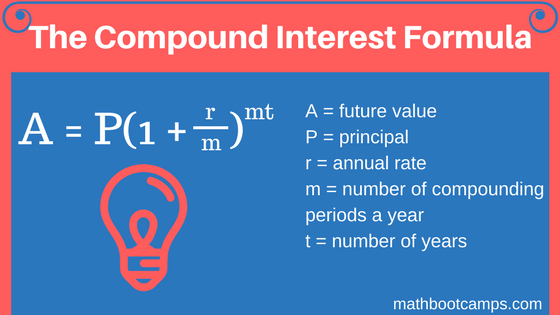﻿ Compound interest formula and examples - MathBootCamps

# Compound interest formula and examples

Compound interest is when interest is earned not only on the initial amount invested, but also on any interest. In other words, interest is earned on top of interest and thus “compounds”. The compound interest formula can be used to calculate the value of such an investment after a given amount of time, or to calculate things like the doubling time of an investment. We will see examples of this below.

## Examples of finding the future value with the compound interest formula

First, we will look at the simplest case where we are using the compound interest formula to calculate the value of an investment after some set amount of time. This is called the future value of the investment and is calculated with the following formula.An investment earns 3% compounded monthly. Find the value of an initial investment of $5,000 after 6 years. ### Solution Determine what values are given and what values you need to find. • Earns 3% compounded monthly: the rate is $$r = 0.03$$ and the number of times compounded each year is $$m = 12$$ • Initial investment of$5,000: the initial amount is the principal, $$P = 5000$$
• 6 years: $$t = 6$$

You are trying to find $$A$$, the future value (the value after 6 years). Now apply the formula with the known values:

\begin{align}A &= P\left(1 + \dfrac{r}{m}\right)^{mt} \\ &= 5000\left(1 + \dfrac{0.03}{12}\right)^{12 \times 6} \\ &\approx \bbox[border: 1px solid black; padding: 2px]{5984.74}\end{align}

### Solution

As before, we are finding the future value, A. In this example, we are given:

• Value after 2 years: $$t = 2$$
• Earns 3% compounded quarterly: $$r = 0.015$$ and $$m = 4$$ since compounded quarterly means 4 times a year
• Principal: $$P = 3500$$

Applying the formula:

\begin{align}A &= P\left(1 + \dfrac{r}{m}\right)^{mt} \\ &= 3500\left(1 + \dfrac{0.015}{4}\right)^{4 \times 2}\\ &\approx \bbox[border: 1px solid black; padding: 2px]{3606.39}\end{align}

### Solution

If we view this as an investment of $$P = 450$$, then we know that the future value is $$A = 750$$. This was after $$t = 10$$ years. Finally, if we assume an annual rate, we will use $$m = 1$$ and have:

$$A = P\left(1 + \dfrac{r}{m}\right)^{mt}$$

$$750 = 450\left(1 + \dfrac{r}{1}\right)^{1 \times 10}$$

This is the same as:

$$750 = 450\left(1 + r\right)^{10}$$

We are solving for the rate, $$r$$. We will do this using the following steps.

Divide both sides by 450.

$$\dfrac{750}{450} = \left(1 + r\right)^{10}$$

Simplify on the left-hand side. But, we need to be careful about rounding, so we will keep the fraction for now.

$$\dfrac{5}{3} = \left(1 + r\right)^{10}$$

Take the left-hand side to the 1/10th power to clear the power of 10 on the right.

$$\left(\dfrac{5}{3}\right)^{\dfrac{1}{10}} = 1 + r$$

Calculate the value on the left and solve for $$r$$.

\begin{align}1.0524 &= 1 + r \\1.0524 – 1 &= r \\ \bbox[border: 1px solid black; padding: 2px] {0.0524} &= r\end{align}

Therefore, Mrs. Jefferson earned an annual rate of 5.24%. Not bad! But there was definitely some more complicated algebra involved. In some cases, you may even have to make use of logarithms. A common situation where you might see this is when calculating the doubling time of an investment at a given rate.

## Calculating the doubling time of an investment using the compound interest formula

Regardless of the amount initially invested, you can find the doubling time of an investment as long as you are given the rate and the number of compounding periods. Let’s look at an example and see how this could be done.

### Example

How many years will it take for an investment to double in value if it earns 5% compounded annually?

It may seem tough to decide where to start here, as we are only given the rate, $$r = 0.05$$, and the number of compounding periods, $$m = 1$$. Note that we are trying to find the time, $$t$$.

Since we do not know the initial investment, we can simply call it $$P$$. For this to double, its value would be $$2P$$ and, using the compound interest formula, we would have:

$$A = P\left(1 + \dfrac{r}{m}\right)^{mt}$$

$$2P = P\left(1 + \dfrac{0.05}{1}\right)^{t}$$

This could be written as:

$$2P = P\left(1.05\right)^{t}$$

Remember that this would only make sense if the amount invested is not zero, so we can divide both side by $$P$$. This gives:

$$2 = \left(1.05\right)^{t}$$

To solve for t, we will take the natural log, ln, of both sides. By the laws of logarithms, this will allow us to bring the exponent to the front.

$$\ln(2) = t\ln\left(1.05\right)$$

Finally, we can divide and then use our calculators to find t.

\begin{align}t &= \dfrac{\ln(2)}{\ln\left(1.05\right)}\\ &\approx \bbox[border: 1px solid black; padding: 2px]{14.2 \text{ years}}\end{align}

Answer: It will take a little more than 14 years before the investment will double in value.

The same process could be used to determine when an investment would triple or even quadruple. You would just use a different multiple of $$P$$ in the first part of the formula.

## Summary

The compound interest formula is used when an investment earns interest on the principal and the previously-earned interest. Investments like this grow quickly; how quickly depends on the rate and the number of compounding periods. When working with a compound interest formula question, always make note of what values are known and what values need to be found so that you stay organized with your work.

Now that you have studied compound interest, you should also review simple interest and how it is different.